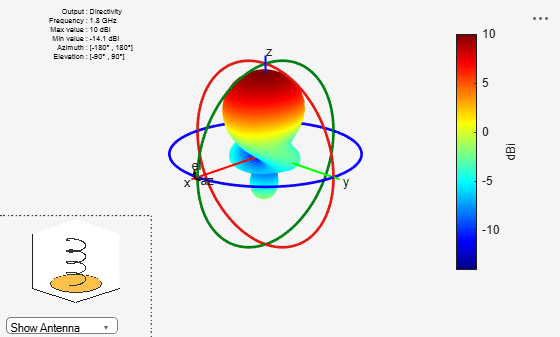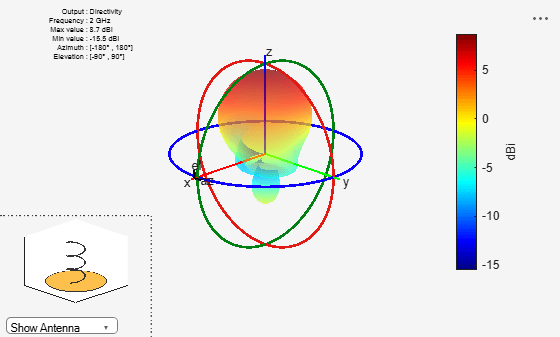Main Content

# helix

Create helix or conical helix antenna on ground plane

## Description

Use the `helix` object to create a helix or conical helix antenna on a circular ground plane. The helix antenna is a common choice in satellite communication.

The width of the strip is related to the diameter of an equivalent cylinder by the equation

`$w=2d=4r$`

where:

• w is the width of the strip.

• d is the diameter of an equivalent cylinder.

• r is the radius of an equivalent cylinder.

For a given cylinder radius, use the `cylinder2strip` utility function to calculate the equivalent width. The default helix antenna is end-fed. The circular ground plane is on the X-Y plane. Commonly, helix antennas are used in axial mode. In this mode, the helix circumference is comparable to the operating wavelength and the helix has maximum directivity along its axis. In normal mode, the helix radius is small compared to the operating wavelength. In this mode, the helix radiates broadside, that is, in the plane perpendicular to its axis. The basic equation for the helix is

$\begin{array}{l}x=r\mathrm{cos}\left(\theta \right)\\ y=r\mathrm{sin}\left(\theta \right)\\ z=S\theta \end{array}$

where

• r is the radius of the helix.

• θ is the winding angle.

• S is the spacing between turns.

For a given pitch angle in degrees, use the `helixpitch2spacing` utility function to calculate the spacing between the turns in meters.Note

In an array of helix antennas, the circular ground plane of the helix is converted to rectangular ground plane.

## Creation

### Syntax

``ant = helix``
``ant = helix(Name,Value)``

### Description

``` `ant = helix` creates a helix antenna operating in axial mode. The default antenna operates around 2 GHz.```

example

````ant = helix(Name,Value)` sets properties using one or more name-value pairs. For example, ```ant = helix('Radius',28e-03)``` creates a helix with turns of radius 28e-03 m.```

### Output Arguments

expand all

Helix antenna, returned as a `helix` object.

## Properties

expand all

Radius of the turns, specified as a positive scalar integer in meters or a two element vector with each element unit in meters. In the two-element vector, the first element specifies the bottom radius and the second element specifies the top radius of the conical helix antenna.

Example: `'Radius',[28e-03 30e-03]`

Example: `ant.Radius = [28e-03 30e-03]`

Data Types: `double`

Strip width, specified as a scalar in meters.

Note

Strip width should be less than `'Radius'`/5 and greater than `'Radius'`/250. 

Example: `'Width',5`

Example: `ant.Width = 5`

Data Types: `double`

Number of turns of the helix, specified as a scalar.

Example: `'Turns',2`

Example: `ant.Turns = 2`

Data Types: `double`

Spacing between turns, specified as a scalar in meters.

Example: `'Spacing',1.5`

Example: `ant.Spacing = 1.5`

Data Types: `double`

Direction of helix turns (windings), specified as `'CW'` or `'CCW'`.

Example: `'WindingDirection'`,`CW`

Example: `ant.WindingDirection = CW`

Data Types: `char` | `string`

Ground plane radius, specified as a scalar in meters. By default, the ground plane is on the X-Y plane and is symmetrical about the origin.

Example: `'GroundPlaneRadius',2.05`

Example: `ant.GroundPlaneRadius = 2.05`

Data Types: `double`

Feeding stub height from ground, specified as a scalar in meters. B

Example: `'FeedStubHeight',2.000e-03`

Example: `ant.FeedStubHeight = 2.000e-03`

Note

The default value is chosen to allow backward compatibility.

Data Types: `double`

Type of the metal used as a conductor, specified as a metal material object. You can choose any metal from the `MetalCatalog` or specify a metal of your choice. For more information, see `metal`. For more information on metal conductor meshing, see Meshing.

Example: ```m = metal('Copper'); 'Conductor',m```

Example: ```m = metal('Copper'); ant.Conductor = m```

Lumped elements added to the antenna feed, specified as a lumped element object handle. You can add a load anywhere on the surface of the antenna. By default, the load is at the origin. For more information, see `lumpedElement`.

Example: `'Load',lumpedelement`. `lumpedelement` is the object handle for the load created using `lumpedElement`.

Example: ```ant.Load = lumpedElement('Impedance',75)```

Data Types: `double`

Tilt angle of the antenna, specified as a scalar or vector with each element unit in degrees. For more information, see Rotate Antennas and Arrays.

Example: `'Tilt',90`

Example: `ant.Tilt = 90`

Example: `'Tilt',[90 90]`,`'TiltAxis',[0 1 0;0 1 1]` tilts the antenna at 90 degrees about the two axes defined by the vectors.

Note

The `wireStack` antenna object only accepts the dot method to change its properties.

Data Types: `double`

Tilt axis of the antenna, specified as:

• Three-element vector of Cartesian coordinates in meters. In this case, each coordinate in the vector starts at the origin and lies along the specified points on the X-, Y-, and Z-axes.

• Two points in space, each specified as three-element vectors of Cartesian coordinates. In this case, the antenna rotates around the line joining the two points in space.

• A string input describing simple rotations around one of the principal axes, 'X', 'Y', or 'Z'.

For more information, see Rotate Antennas and Arrays.

Example: `'TiltAxis',[0 1 0]`

Example: `'TiltAxis',[0 0 0;0 1 0]`

Example: `ant.TiltAxis = 'Z'`

Note

The `wireStack` antenna object only accepts the dot method to change its properties.

Data Types: `double`

## Object Functions

 `show` Display antenna or array structure; display shape as filled patch `info` Display information about antenna or array `axialRatio` Axial ratio of antenna `beamwidth` Beamwidth of antenna `charge` Charge distribution on metal or dielectric antenna or array surface `current` Current distribution on metal or dielectric antenna or array surface `design` Design prototype antenna or arrays for resonance at specified frequency `efficiency` Radiation efficiency of antenna `EHfields` Electric and magnetic fields of antennas; Embedded electric and magnetic fields of antenna element in arrays `impedance` Input impedance of antenna; scan impedance of array `mesh` Mesh properties of metal or dielectric antenna or array structure `meshconfig` Change mesh mode of antenna structure `optimize` Optimize antenna or array using SADEA optimizer `pattern` Radiation pattern and phase of antenna or array; Embedded pattern of antenna element in array `patternAzimuth` Azimuth pattern of antenna or array `patternElevation` Elevation pattern of antenna or array `returnLoss` Return loss of antenna; scan return loss of array `sparameters` S-parameter object `vswr` Voltage standing wave ratio of antenna

## Examples

collapse all

Create and view a helix antenna that has a 28 mm turn radius, 1.2 mm strip width, and 4 turns.

`hx = helix('Radius',28e-3,'Width',1.2e-3,'Turns',4)`
```hx = helix with properties: Radius: 0.0280 Width: 0.0012 Turns: 4 Spacing: 0.0350 WindingDirection: 'CCW' FeedStubHeight: 1.0000e-03 GroundPlaneRadius: 0.0750 Conductor: [1x1 metal] Tilt: 0 TiltAxis: [1 0 0] Load: [1x1 lumpedElement] ```
`show(hx)`Plot the radiation pattern of a helix antenna.

```hx = helix('Radius',28e-3,'Width',1.2e-3,'Turns',4); pattern(hx,1.8e9);```Calculate the spacing of a helix that has a pitch of 12 degrees and a radius that varies from 20 mm to 22 mm in steps of 0.5 mm.

`s = helixpitch2spacing(12,20e-3:0.5e-3:22e-3)`
```s = 1×5 0.0267 0.0274 0.0280 0.0287 0.0294 ```

Plot the radiation pattern of a helix antenna with transparency specified as 0.5.

`p = PatternPlotOptions`
```p = PatternPlotOptions with properties: Transparency: 1 SizeRatio: 0.9000 MagnitudeScale: [] AntennaOffset: [0 0 0] ```
```p.Transparency = 0.5; ant = helix; pattern(ant,2e9,'patternOptions',p)```To understand the effect of Transparency, chose `Overlay Antenna` in the radiation pattern plot.

This option overlays the helix antenna on the radiation pattern.Balanis, C.A. Antenna Theory. Analysis and Design, 3rd Ed. New York: Wiley, 2005.

 Volakis, John. Antenna Engineering Handbook, 4th Ed. New York: Mcgraw-Hill, 2007.

 Zhang, Yan, Q. Ding, J. Chen, S. Lu, Z. Zhu and L. L. Cheng. “A Parametric Study of Helix Antenna for S-Band Satellite Communications.” 9th International Symposium on Antenna Propagation and EM Theory (ISAPE). 2010, pp. 193–196.

 Djordjevic, A.R., Zajic, A.G., Ilic, M. M., Stuber, G.L. “Optimization of Helical antennas (Antenna Designer's Notebook)” IEEE Antennas and Propagation Magazine. December, 2006, pp. 107, pp.115.

## Support

#### Hybrid Beamforming for Massive MIMO Phased Array Systems

Download the white paper